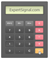Commodity Channel Index

(CCI)

Usage: Identifying Overbought/Oversold Markets

Standard Settings: 14 Periods (Recommended 20 Periods)Introduction to CCI

The Commodity Channel Index or CCI is an oscillator that aims to identify overbought/oversold market levels. CCI is able to measure the current position of a financial asset with respect to its moving average. CCI can also signal trades as you can see below, these signals are more reliable in ranging markets.Calculating CCI

CCI = (TP - SMA (20) of TP) / (Constant x Mean Deviation)

Where:

• TP (Typical Price) = (High + Low + Close) / 3
• Constant = .015

Mean Deviation can be calculated as follows:

(i) The 20-period average of the Typical Price is subtracted from each period's Typical Price

(ii) The absolute values of these numbers are taken

(iii) The absolute values are summed

(iv) The total number is divided by the total number of periods. In our case by 20 periodsTrading with CCI

CCI can be used for multiple purposes:

• Identifying Overbought/Oversold Markets
• Trading Signals on Slopes Divergences (price chart slope vs CCI slope)

The CCI can generate buy/sell trading signals -The crucial points are -100 and +100.

When CCI is found below -100 and suddenly moves above -100 you get a buy signal

When CCI is found above +100 and suddenly moves below +100 you get a sell signal

Notes:

-CCI is more effective in ranging markets than in trending markets

-Furthermore, the signals are more reliable when CCI has spent a number of time periods above +100 in case of selling signals or below -100 in case of buying signals

-Finally, when a divergence between the slope of the price chart and the CCI chart occurs then CCI can offer even more powerful trading signals. That divergence between the CCI and the price chart may be either bullish or bearishPlatform Setup

□ GO TO → INDICATORS → OSCILLATORS → COMMODITY CHANNEL INDEX

□ STANDARD SETTINGS → 14 PERIODS

Commodity Channel Index (CCI)